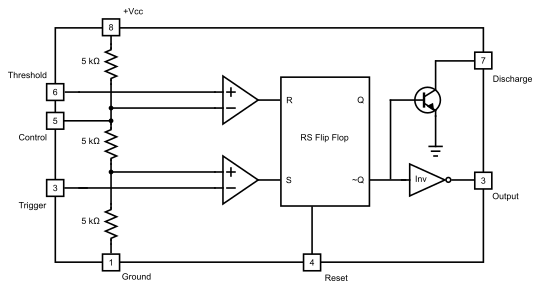# 555 timer

Martin McBride, 2020-05-31
Tags 555 timer
Categories electronics analogue electronics analogue electronic components

The NE555 timer is a popular chip that can be used either as a timer or a square wave oscillator. It usually only requires a few passive components.

## Characteristics

The chip has the following characteristics:

• Supply voltage between 4.5V and 16V.
• Output can source or sink up to 200mA.
• Timer ranging from 10uS to several hours.
• Oscillator frequency from 100KHz to less than 1 cycle per hour.
• Can generate square wave or approximate saw wave.
• Variable duty cycle (on time vs off time).
• Frequency of the oscillator can be voltage controlled.

There are numerous variants of the 555 timer that slightly different characteristics. Check the data sheet of the actual device you are using.

## Oscillator operation

The 555 is often used as an oscillator. It can operate over the full audio range, and can also be used as a low frequency oscillator, including very, very low frequencies (it can oscillate with cycle times of longer than one hour, although for musical use you would rarely need such a long cycle time).

The frequency and duty cycle of the oscillator is determined by a capacitor and two resistors. By substituting potentiometers for the resistors, the frequency and duty cycle can be varied.

A control voltage can be used to vary the frequency of the oscillator. However, the result will not be linear or follow a 1V per octave rule.

The output of the oscillator is a square wave. It can source or sink up to 200mA, but the chip will run hot at those loads. The output voltage will switch between a low value (typically about 0.1V, assuming a 10mA load) and a high value (typically equal to the supply voltage minus 1.5V, again with a 10mA load).

The voltage at the capacitor of the oscillator can be used to derive and approximately triangular/sawtooth wave/

## Timer operation

The 555 can also be used in a monostable (one shot) mode. In that case, an input trigger pulse sets the output high. The output then remains high for a fixed period, then returns to a low value. It remains low indefinitely, until it receives another input pulse.

This signal can be used as a simple envelope, that allows gates signal for a certain length of time. The time period is determined by a resistor and capacitor, and like the oscillator case, the time length can be made variable using a potentiometer, or can be voltage controlled.

The signal at the capacitor rises from 0 to about two thirds of the supply voltage during the on period. This can be used as an attacl envelope. Several 555 timers can be used to create more complex envelopes such as an ADSR envelope.

## Pulse width modulation

The 555 has many applications, but as a final example, it can be used as a pulse width modulator. This a a circuit that produces a square wave whose pulse width is proportional to the value of a control voltage. This allows an analogue signal to be converted into a digital signal (that has a value on or off). The signal can be converted back into an analogue signal by finding its average voltage over each cycle.

## 555 internals

The 555 chip internals are clever, but relatively simple. It consists of two comparators, a voltage divider, and a flip-flop, plus a few extra components: